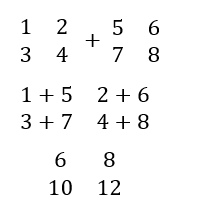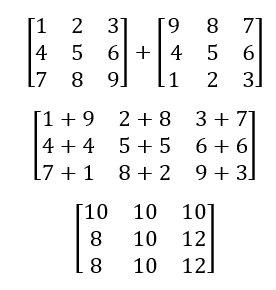How Do You Add Matrices? Operations on matrices are one of the easiest concepts you'll learn in mathematics. However, similar to other core concepts, the calculation needs to be precise, where there is no margin for error. Now, if you want to add two matrices, the method is quite conventional, without any prerequisite conditions. In other words, you can just add the first element of the first matrix with the first element of the second and move on to the rest of them. Let us consider adding the following two matrices.Let us take another example, where we have two 3 x 3 matrices:• ### Basic Lesson

Guides students through solving the sum of matrices. If A and B are two matrices each of order m X n, then their sum A + B is a matrix of order m X n and is obtained by adding the corresponding elements of A and B.

• ### Intermediate Lesson

Demonstrates how to calculate the sum of three row-column matrices. 1. Add the first element of the first table with the first element of the second table. 2. Accordingly, add the second element of the first table with the second element of the second table. 3. Perform addition of all the corresponding elements.

• ### Independent Practice 1

A really great activity for allowing students to understand the concept of Addition of Matrices.

• ### Independent Practice 2

Students find the Addition of Matrices in assorted problems. The answers can be found below.

• ### Homework Worksheet

Students are provided with problems to achieve the concepts of Addition of Matrices.

• ### Skill Quiz

This tests the students ability to evaluate Addition of Matrices.

• ### Homework and Quiz Answer Key

Answers for the homework and quiz.